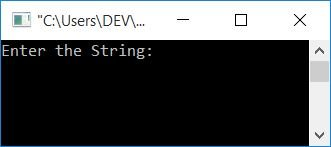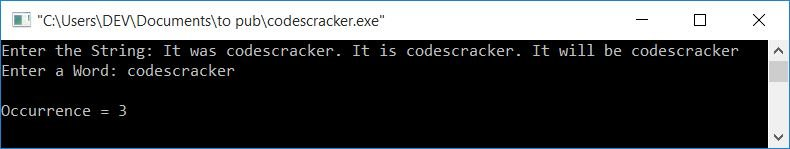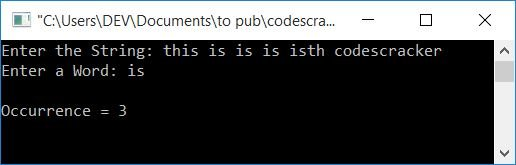# C Program to Count the Occurrences of a Word in a String

This article will teach you and provide you with code to count the occurrences of a word in a string using the C programming language. The user must enter the word and string here (at run-time). For example, if the given string is:

`It was codescracker. It is codescracker. It will be codescracker`

then the occurrence of the word

• codescracker is 3
• It is 3
• was is 1
• is is 1
• will is 1
• be is 1

Now let's move on and implement it in a C program.

## Counting Words in a String

To count the occurrence of a word in a string or sentence in C programming, you have to ask the user to enter the string and a word, and then count how many times the given word is available in the given string, as shown in the program given below:

```#include<stdio.h>
#include<conio.h>
#include<string.h>
int main()
{
char str, word;
int i, j, ls, lw, temp, countW=0, chk;
printf("Enter the String: ");
gets(str);
printf("Enter a Word: ");
gets(word);
ls = strlen(str);
lw = strlen(word);
for(i=0; i<ls; i++)
{
temp = i;
for(j=0; j<lw; j++)
{
if(str[i]==word[j])
i++;
}
chk = i-temp;
if(chk==lw)
countW++;
i = temp;
}
printf("\nOccurrence = %d", countW);
getch();
return 0;
}```

The program was written in the Code::Blocks IDE. Here is its sample run after a successful build and run:Now supply any string, say

`It was codescracker. It is codescracker. It will be codescracker`

And then enter a word, say "codescracker," to count how many times this word occurred in the given string:#### Program Explained

• The two variables, ls and lw, are used to hold the length of string and word, respectively.
• The first for loop runs from the first character to the last character of the string.
• And the second for loop runs from the first character to the last character of the given word.
• In the second for loop, we have checked whether the given word is present inside the string or not, using character-by-character matching.
• That is, if the first character of a word gets found in the string at any index, then it checks for a second match. That is, match the second character of a word with the character just after the previous index, and so on.
• For example, let's suppose the given word is "codescracker." So its length is 12. Therefore, if all characters continuously get matched in the given string, then the value of i will be (its previous value + 12).
• And after exiting the second for loop, using i-temp or (i's previous value + 12)-temp gets initialized to chk.
• If the previous value of i was 7, the value of i will be 7 + 12 or 19.
• And 19-temp or 19-7 equals 12. As a result, 12 is set up to chk.
• Now check whether the value of chk is equal to the length of the word or not.
• If it is equal, then the word gets found, so we have to increment the value of the countW variable, which holds the occurrence of the word.
• Finally, initialize the value of temp (the value of the previous i before the second for the loop).
• And so on until you reach the last character of the string.

This program has a limitation: if you enter a string, such as "this is codescracker," and a word, such as "is," the occurrence will be displayed as 2. Because "is" from "this" will be counted as well. To overcome this problem, let me create another program for you.

### Complete Version of the Counting a Word in a String Program

Here is the complete version of the previous program:

```#include<stdio.h>
#include<conio.h>
#include<string.h>
int main()
{
char str, word;
int i, j, ls, lw, temp, countW=0, chk, doIncrement;
printf("Enter the String: ");
gets(str);
printf("Enter a Word: ");
gets(word);
ls = strlen(str);
lw = strlen(word);
for(i=0; i<ls; i++)
{
temp = i;
doIncrement = 0;
for(j=0; j<lw; j++)
{
if(str[i]==word[j])
{
if(temp>0 && (temp+lw)<ls)
{
if(str[temp-1]== ' ' && str[temp+lw]==' ')
doIncrement=1;
}
else if(temp==0 && (temp+lw)<ls)
{
if(str[temp+lw]==' ')
doIncrement=1;
}
else if(temp>0 && (temp+lw)==ls)
{
if(str[temp-1]== ' ')
doIncrement=1;
}
if(doIncrement==1)
i++;
else
break;
}
}
chk = i-temp;
if(chk==lw)
countW++;
i = temp;
}
printf("\nOccurrence = %d", countW);
getch();
return 0;
}```

Now let's check it out with string input as "this is is is isth codescracker," and word input as "is."#### The same program in different languages

C Quiz

« Previous Program Next Program »